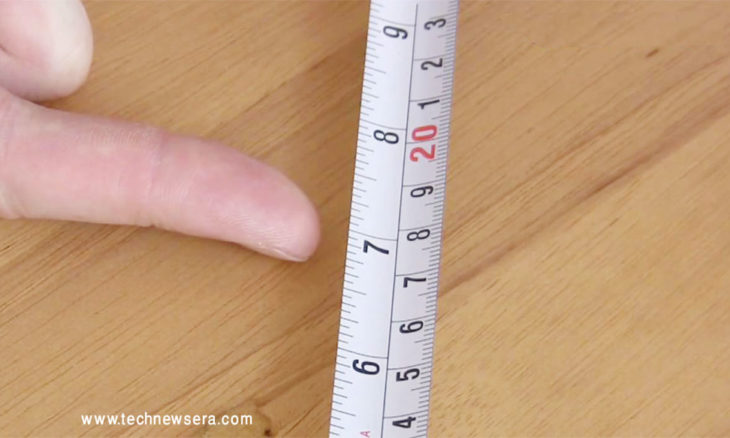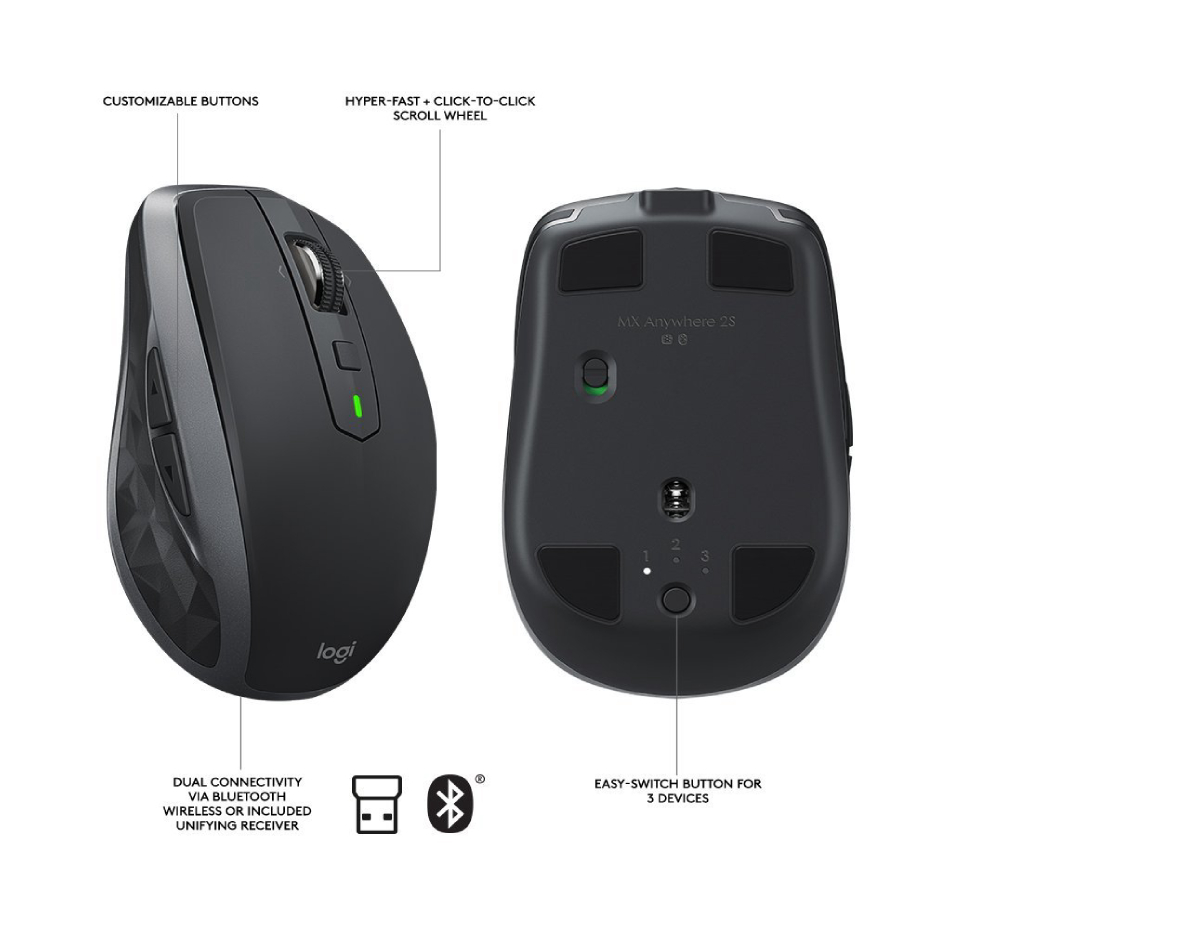# Conversion of Millimeters to InchesOver the world, there are different ways to measure things. There are different units for each measurement, and each place we go to has its own unit of measurement. Today we will discuss Conversion of Millimeters to Inches.

To keep up with these different units we convert them into each other, some of the common conversions are as below:

• Yards to inches
• Yards to miles
• Inches to yards
• Miles to kilometers
• Kilometers to yards
• Miles to yards

To clear out the confusion that comes with all these units of measurement, a new system was introduced that standardized the units and defined the basic unit according to which we now measure and according to which we convert the units.

In 1960, we were given the international system of units or more commonly known as the SI unit to standardize a single unit and make it a reference for all other conversions. This system gave us a standardized unit to use.

Millimeter and inches are both unit of measuring length, millimeter is a standardized unit of measurement according to the SI unit the inch is mostly used in the USA.

## Millimeters:

A milli meter is a relatively smaller unit of displacement (that can be length or distance) according to the international system of unit hence making it a standardized unit of measurement for displacement for science, engineering, technology and mathematics.

The word meter is taken from the Greek language and translate to “Measure”. This unit is used to measure very small but visible distances and lengths. In comparison to the real world, a millimeter is approximately the size of the wire used in making paper clips.

Must Know:  4 Hydraulic Pump Technology and Market Trends to Keep an Eye On

The millimeter is denoted by “MM” and is the smaller unit of centimeter and meter:

• There are 10 mm in 1 cm
• There are 1000 mm in 1 m

Meter is the actual unit and millimeter is its variant and along with milli meter here are some of its other variants:

• A nanometer is a one billionth part of a meter
• A micrometer is a one millionth part of a meter
• A millimeter is a one thousandth part of a meter
• A centimeter is one hundredth part of a meter
• A decimeter is one tenth part of a meter

## Inches:

The inch is a unit of length mostly used by the United States and the British customary systems of measurement. It equals to a 36th part of a yard or a 12th part of a foot. This word was taken from the Latin word of “Uncia”, which is not present in other Germanic languages.

The word inch also translates to some similar units which are used in other measurement systems. It is generally described as being equal to approximately the width of a human thumb. The exact length of an inch has varied in the past but since the SI system has been introduced it has been defined to be 2.54 cm.

The inch is mostly used in:

• United Kingdom
• United States
• Japan
• Continental Europe (mostly just for the size of display screens)

## Conversion of millimeters to inches:

Now as we learned that the inch is equal to 2.54 cm and 1 cm has 10 mm that means we can calculate that 1 inch is equal to 25.4 mm.

The conversion of millimeters to inches can be easily understood by the fraction method which makes it simple and relatively easy to calculate without the help of any calculator or website.

Must Know:  The future of Virtual Reality Technology 2017

The numerator and the denominator are the same lengths written in different units so that the fraction equals to 1 for e.g. if 1 inch is the nominator than 25.4 mm will be the denominator so that the resulting answer be 1.

### Millimeter (MM) to Inch Conversion Chart.

 Millimeter [mm] Inch [in] 0.01 -mm 0.0003937008 in 0.1 -mm 0.0039370079 in 1 -mm 0.0393700787 in 2 -mm 0.0787401575 in 3 -mm 0.1181102362 in 5 -mm 0.1968503937 in 10 -mm 0.3937007874 in 20 -mm 0.7874015748 in 50 -mm 1.968503937 in 100 -mm 3.937007874 in 1000 -mm 39.3700787402 in

Now multiply this fraction with the length you want to measure. If you have set up your fraction right the same units will be canceled by each other and you will be left with your desired unit and after that, you just have to solve the fraction and you will have your answer in your desired unit.